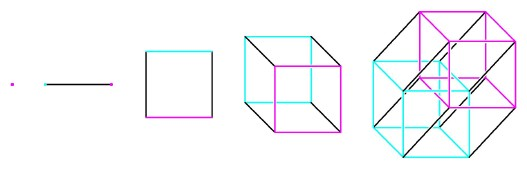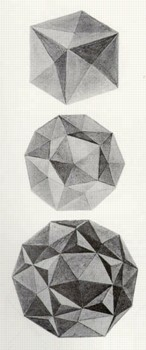Dimensional Progressions

Dimensional analogies did not begin with Flatland. The power of comparing insights from different dimensions already occurs explicitly in the works of Plato. In the seventh book of the Republic, Socrates converses with Glaucon about the education of the guardians of an ideal state: start with arithmetic and the study of the number line, then proceed to plane geometry, an essential understanding for anyone charged with military defense or the layout of cities. When Socrates asks what should come next, Glaucon suggests astronomy. Socrates chides him for missing an essential step, that of solid geometry, a subject that he considers to be neglected in the schools of his day. Only after proceeding from the first dimension to the second and then to the third would a student be prepared to consider the motions of the heavens.

Plato recognized the built-in progression of dimensions, and he knew how powerful the devices of analogy could be in suggesting solid geometry theorems corresponding to results in plane geometry. But strictly speaking, he did not let the momentum of one to two to three carry him into a spatial fourth dimension. The response to that invitation came only many centuries later, in the early 1800s, when mathematicians in different parts of the world opened their minds to new kinds of geometry. One breakthrough was the discovery of non-Euclidean geometries, satisfying all but one of Euclid's plane geometry axioms. Another crucial insight occurred when mathematicians realized that our plane and solid geometries were just the beginning of a sequence of geometries of higher and higher dimension. Both of these developments challenged the prevailing view that geometry was totally confined to the description of direct physical experience. Inability to visualize what non-Euclidean and higher-dimensional geometries might mean caused many to reject them. Writers like Abbott and Karl Friedrich Gauss and Hermann von Helmholz developed dimensional analogies to enlarge the faculty of imagination so that people could consider the new mathematical creations.

Analogy is surely the dominant idea in the history of the concept of dimensions. If we truly understand a theorem in plane geometry, then we should be able to find one or more analogies in solid geometry, and conversely, solid geometry theorems will often suggest new relationships among plane figures. Theorems about squares should correspond to theorems about cubes or square prisms. Theorems about circles should be analogous to theorems about spheres or cylinders or cones. But if we learn a good deal by going from two dimensions to three, would we not learn even more by going from three dimensions to four?The analogue of a cube in any dimension can be generated by moving the preceding lower-dimensional cube perpendicular to itself.In an 1880 paper, William Stringham used techniques from analytical geometry to produce illustrations showing partially completed, regular four-dimensional figures projected into three-dimensional space.

Mathematicians began to follow different paths within this progression, developing sequences of analogous figures starting even farther back along the dimensional ladder. One possible sequence started with a point, having zero dimensions, no degrees of freedom A point moving in a straight line generates a segment with two endpoints, a fundamental one-dimensional object. A segment moving perpendicular to itself in a plane generates a figure with four corners, a square, the basic object in the second dimension. Proceeding to the third dimension, we move a square perpendicular to itself to form a cube, a basic three-dimensional object. Even though A Square could no longer appreciate this process fully, he could follow along at a theoretical level and deduce certain properties of this cube that he could not see — for example, that it has eight corners. Next we ask what appears if we move a cube in a fourth direction perpendicular to all its edges. We would get a basic four-dimensional object, a hypercube, and although we can no longer fully appreciate the process, we can predict that such a hypercube will have 16 corners. The numbers of corner points generate a geometric progression, and we can easily arrive at a formula for the number of corners of a cube in any dimension.

A consideration of boundaries leads to another progression. A segment has two boundary points. A square is bounded by four segments. The boundary of a cube is six squares. Following this progression, we expect that the hypercube will be bounded by cubes, and that there will be eight of them. The formula for the number of boundary pieces in any dimension can be found by using an arithmetic progression.

But does a hypercube really exist? Mathematicians did not feel they had to answer that question. They could predict the numbers of corners and boundary pieces of analogues of the cube in any dimension, whether or not they corresponded to some physical reality. However, these lists of numbers still left something to be desired. In plane and solid geometry, the objects were not only real, they could also be represented as diagrams or models, capable of revealing significant relationships. How can we see what a hypercube would look like? If we can't see it, how do we know that our assertions about it are true?

Geometers in the last century devised methods for visualizing objects in higher dimensions, and these methods are worth looking at over the course of this book. Quite sophisticated in some ways but with limitations that were often frustrating, the imaging and modeling techniques of a hundred years ago were inadequate for interpreting complicated objects in four dimensions. Eventually higher-dimensional geometry came to be based not only on analogy but on coordinate geometry, which could translate geometric concepts into numerical and algebraic form. Although such formal methods put the mathematics on a firm footing, they could not satisfy the desire to "see" the higher dimensions.

For the task of visualizing objects and relationships in the fourth and higher dimensions, the ideal instrument is the modern graphics computer.